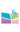﻿2022.9.27，童平，副教授, ​Nanyang Technological University (NTU) Singapore，Key roles of mathematics in seismic imaging, medical imaging and non-destructive testing-中国矿业大学（北京）理学院网站2022.9.27，童平，副教授, ​Nanyang Technological University (NTU) Singapore，Key roles of mathematics in seismic imaging, medical imaging and non-destructive testing

Given an n by n matrix A and an n by 1 vector a, the process of computing Aa is to solve a forward problem. On the contrary, given an n by n matrix A and an n by 1 vector b, the process of solving Ax=b for the vector x is to solve an inverse problem. Simply speaking, solving an inverse problem is to find the causing factor x from observation b. Mathematical inverse theory is at the core of many disciplines, including frequently mentioned medical imaging, seismic imaging, and non-destructive testing. A typical inverse problem consists of four aspects: (1) a mathematical physical model to describe a particular phenomenon, such as eikonal equations and acoustic/elastic wave equations used to model seismic wave propagation, photoacoustic equation adopted to simulate the interaction between photoacoustic signals and human bodies; (2) an efficient numerical algorithm to solve the corresponding equation; (3) a reliable scheme to determine the causing factors (usually the parameters of a partial differential equation) from observations; (4) Interpreting the results. In the talk, I will mainly show how mathematical theory and numerical methods are used to locate earthquakes, delineate crust and mantle structures, detect underground bedrock, holes, tunnels and some others. Applications in medical imaging and non-destructive testing will also be discussed.

Ping Tong is an associate professor holding a joint position with the Division of Mathematical Sciences and Asian School of the Environment at Nanyang Technological University (NTU) Singapore. He is also a principal investigator at Earth Observatory of Singapore. Ping got his Ph.D. in computational mathematics from Tsinghua University in 2012. Before joining NTU in September 2016, he was a postdoctoral fellow at University of Toronto (2012-2014) and Stanford University (2014-2016). He is supervising 8 post-doctoral scholars and 3 PhD students. He has published more than 50 papers in high profile journals, including GRL, JGR, GJI, EPSL, etc. His research fields include computational mathematics (inverse problems and numerical solutions of partial differential equations), seismic imaging and medical imaging.